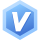# 关于#权值#的问题，如何解决？(语言-python)

【每日一练】—— 吃!吃!吃!_已知n个整数。 每3个数算一个周期。 不足一个周期补0。 在周期的第一个位置的数的权值和等于a1+a[1+3]+… … 求三个数的最大权值。 如果最大权值为第一个数，输出’J’； 最大权值为第二个数，输出’H’； 最大权值为第三个数，输出’B’。 输入描述： 第一行输入一个整数n。(1<=n<=100) 第二行输入n个整数。 输出描述： 输出答案。 输入样例： 4 1 1 1 1 输出样例： J ```python class Solution: def __init__(self): pass def solution(self, n, arr): a = [] if n % 3 != 0: for i in range(n, (int(n / 3) + 1) * 3): arr.append(0) n +=1 for i in range(0, 3): sum_i = 0 for j in range(0, int(n / 3)): sum_i += arr[i + 3 * j] a.append(sum_i) if a.index(max(a)) == 0: result = "J" elif a.index(max(a)) == 1: result = "H" else: result = "B" return result if __name__ == "__main__": n = int(input().strip()) arr = [int(item) for item in input().strip().split()] sol = Solution() result = sol.solution(n, arr) print(result) ```
• 写回答

#### 1条回答默认 最新

•关注
评论

#### 悬赏问题

• ¥30 关于#开发语言#的问题：我需要在抄板的基础上再抄板抄程序，根据RDA8851CM基础上开发
• ¥15 oracle 多个括号,怎么删除指定的括号及里面的内容?
• ¥15 poly函数表达式的看法
• ¥15 我想问一下有人熟悉思科packet tracer
• ¥15 小新14API2019想用bios调风扇
• ¥15 Python和易语言爬虫探讨
• ¥15 有Sakai 会吗，没做过，需要交
• ¥20 使用vba登录公司统一登录的网页
• ¥15 opensea上传人机验证不了
• ¥15 RK3568 kernel5.10